# MobileNetv1 论文详解

MobileNet 论文的主要贡献在于提出了一种深度可分离卷积架构（DW+PW 卷积），先通过理论证明这种架构比常规的卷积计算成本（Mult-Adds）更小，然后通过分类、检测等多种实验证明模型的有效性。

## 1、相关工作

### 标准卷积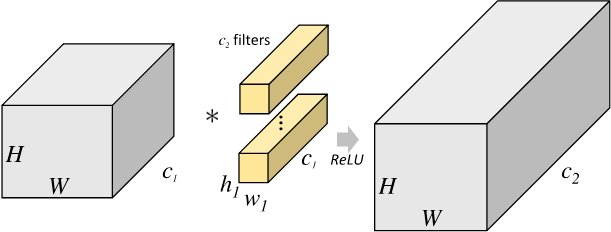2D 卷积计算过程动态图如下，通过这张图能够更直观理解卷积核如何执行滑窗操作，又如何相加并输出 $c_2$ 个 feature map ，动态图来源 这里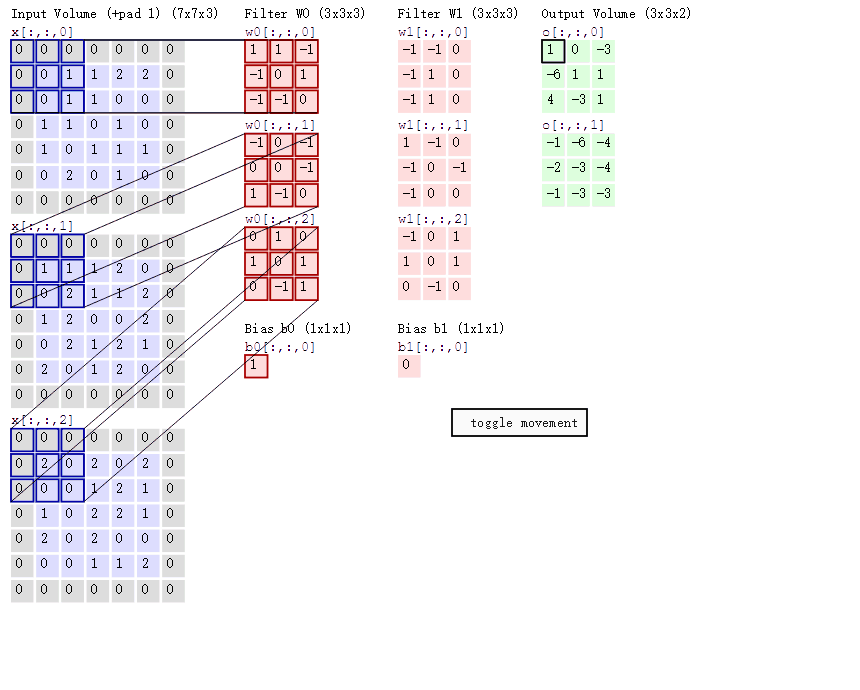### 分组卷积

Group Convolution 分组卷积，最早见于 AlexNet。常规卷积与分组卷积的输入 feature map 与输出 feature map 的连接方式如下图所示，图片来自CondenseNet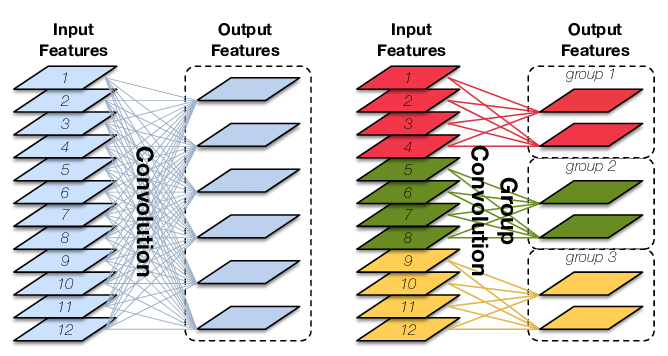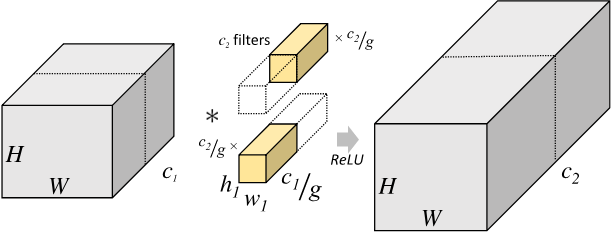\begin{aligned} & MACC = H \times W \times 1 \times 1 \times \frac{c_{1}}{g}\frac{c_{2}}{g} \times g = \frac{hwc_{1}c_{2}}{g} \ & FLOPs = 2 \times MACC \ & Params = g \times \frac{c_2}{g}\times\frac{c_1}{g} \times 1\times 1 + c_2 = \frac{c_{1}c_{2}}{g} \ & MAC = HW(c_1 + c_2) + \frac{c_{1}c_{2}}{g} \ \end{aligned}

### 从 Inception module 到 depthwise separable convolutions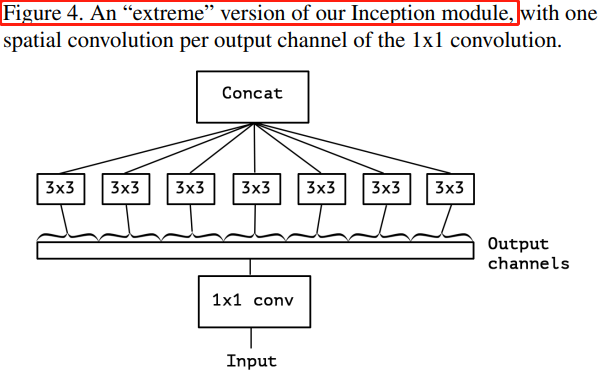Figure 4 中的“极限” Inception 模块与本文的主角-深度可分离卷积模块相似，区别在于：深度可分离卷积先进行 channel-wise 的空间卷积，再进行 $1 \times 1$ 的通道卷积，Figure 4 的 Inception 则相反；

## 2、MobileNets 结构

### 2.1，深度可分离卷积

MobileNets 是谷歌 2017 年提出的一种高效的移动端轻量化网络，其核心是深度可分离卷积（depthwise separable convolutions），深度可分离卷积的核心思想是将一个完整的卷积运算分解为两步进行，分别为 Depthwise Convolution（DW 卷积） 与 Pointwise Convolution（PW 卷积）。深度可分离卷积的计算步骤和滤波器尺寸如下所示。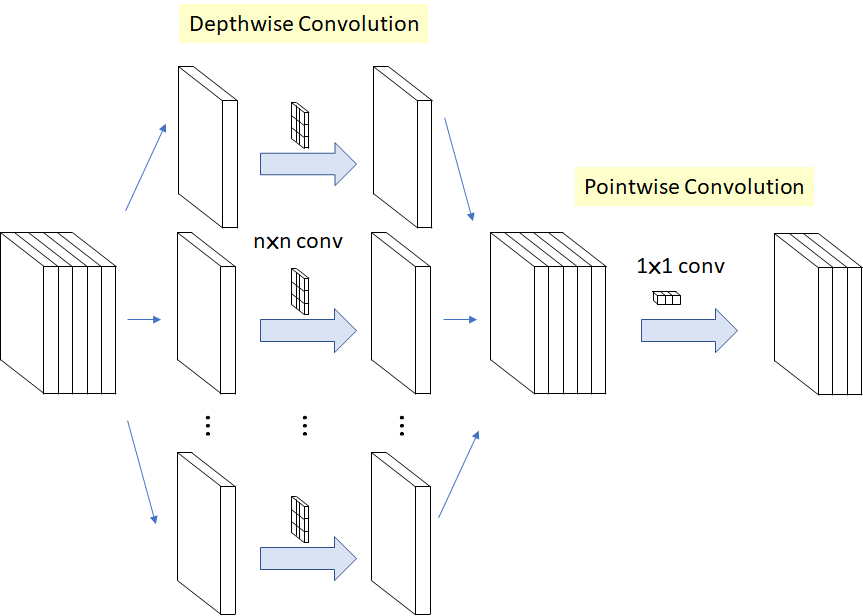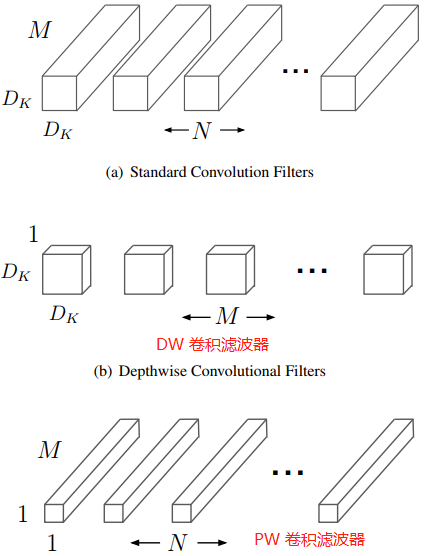#### Depthwise 卷积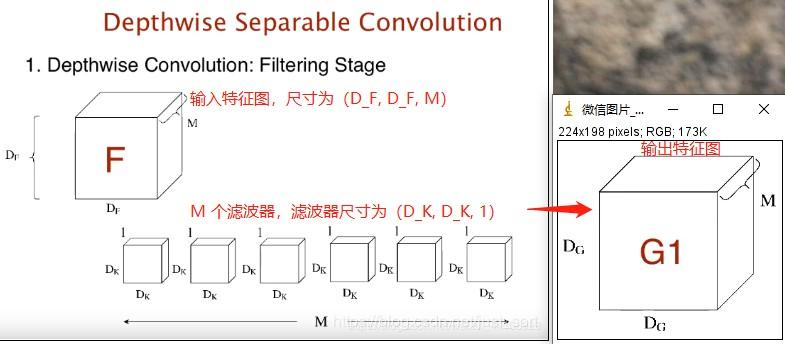DW 卷积的计算量 $MACC = M \times D_{G}^{2} \times D_{K}^{2}$

#### Pointwise 卷积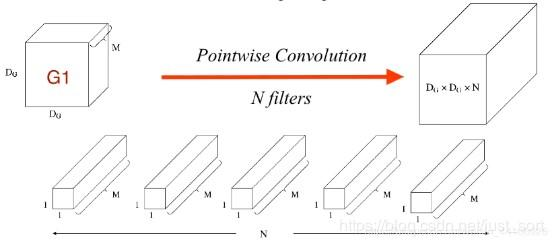\begin{aligned} \frac{Depthwise \ Separable \ Conv}{Standard \ Conv} &= \frac{M \times D_{G}^{2}(D_{K}^{2} + N)}{N \times D_{G}^{2} \times D_{K}^{2} \times M} \ &= \frac{D_{K}^{2} + N}{D_{K}^{2} \times N} \ &= \frac{1}{N} + \frac{1}{D_{K}^{2}} \ \end{aligned}

### 2.2、网络结构

$3 \times 3$ 的深度可分离卷积 Block 结构如下图所示：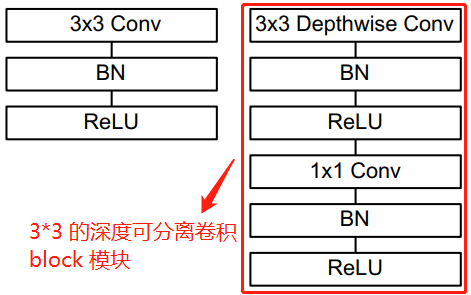python class MobilnetV1Block(nn.Module): """Depthwise conv + Pointwise conv""" def init(self, in_channels, out_channels, stride=1): super(MobilnetV1Block, self).init() # dw conv kernel shape is (in_channels, 1, ksize, ksize) self.dw = nn.Conv2d(in_channels, in_channels, kernel_size=3,stride=stride,padding=1, groups=in_channels, bias=False) self.bn1 = nn.BatchNorm2d(in_channels) self.pw = nn.Conv2d(in_channels, out_channels, kernel_size=1, stride=1, padding=0, bias=False) self.bn2 = nn.BatchNorm2d(out_channels)

def forward(self, x):
out1 = F.relu(self.bn1(self.dw(x)))
out2 = F.relu(self.bn2(self.pw(out1)))
return out2




MobileNet v1pytorch 模型导出为 onnx 模型后，深度可分离卷积 block 结构图如下图所示。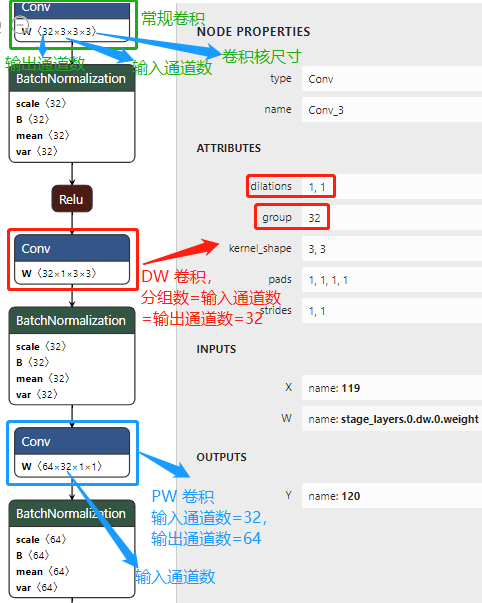MobileNet 模型结构将几乎所有计算都放入密集的 1×1 卷积中（dense 1 × 1 convolutions），卷积计算可以通过高度优化的通用矩阵乘法（GEMM）函数来实现。 卷积通常由 GEMM 实现，但需要在内存中进行名为 im2col 的初始重新排序，然后才映射到 GEMM。 caffe 框架就是使用这种方法实现卷积计算。 1×1 卷积不需要在内存中进行重新排序，可以直接使用 GEMM（最优化的数值线性代数算法之一）来实现。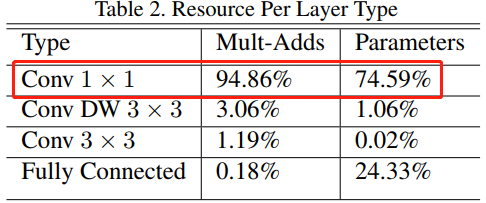### 2.3、宽度乘系数-更小的模型

$$\alpha M \times D_{G}^{2} \times D_{K}^{2} + \alpha N \times \alpha M \times D_{G}^{2}$$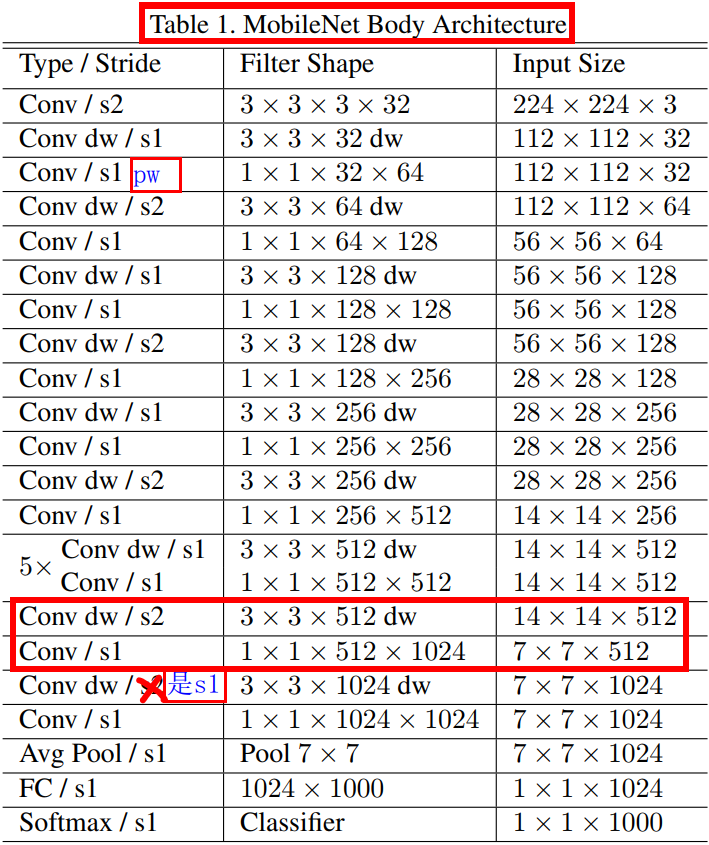### 2.5、模型结构总结

• 整个网络不算平均池化层与 softmax 层，且将 DW 卷积和 PW 卷积计为单独的一层，则 MobileNet28 层网络。+ 在整个网络结构中步长为2的卷积较有特点，卷积的同时充当下采样的功能；
• 第一层之后的 26 层都为深度可分离卷积的重复卷积操作，分为 4 个卷积 stage
• 每一个卷积层（含常规卷积、深度卷积、逐点卷积）之后都紧跟着批规范化和 ReLU 激活函数；
• 最后一层全连接层不使用激活函数。

## 5、基准模型代码

python import torch import torch.nn as nn import torch.nn.functional as F import torchvision.models as models from torch import flatten

class MobilnetV1Block(nn.Module): """Depthwise conv + Pointwise conv"""

def __init__(self, in_channels, out_channels, stride=1):
super(MobilnetV1Block, self).__init__()
# dw conv kernel shape is (in_channels, 1, ksize, ksize)
self.dw = nn.Sequential(
nn.Conv2d(in_channels, 64, kernel_size=3,
nn.BatchNorm2d(in_channels),
nn.ReLU(inplace=True)
)
# print(self.dw.weight.shape)  # print dw conv kernel shape
self.pw = nn.Sequential(
nn.Conv2d(in_channels, out_channels, kernel_size=1,
nn.BatchNorm2d(out_channels),
nn.ReLU(inplace=True)
)

def forward(self, x):
x = self.dw(x)
x = self.pw(x)
return x


def convbn_relu(in_channels, out_channels, stride=2): return nn.Sequential(nn.Conv2d(in_channels, out_channels, kernel_size=3, stride=stride, padding=1, bias=False), nn.BatchNorm2d(out_channels), nn.ReLU(inplace=True))

class MobileNetV1(nn.Module): # (32, 64, 1) means MobilnetV1Block in_channnels is 32, out_channels is 64, no change in map size. stage_cfg = [(32, 64, 1), (64, 128, 2), (128, 128, 1), # stage1 conv (128, 256, 2), (256, 256, 1), # stage2 conv (256, 512, 2), (512, 512, 1), (512, 512, 1), (512, 512, 1), (512, 512, 1), (512, 512, 1), # stage3 conv (512, 1024, 2), (1024, 1024, 1) # stage4 conv ] def init(self, num_classes=1000): super(MobileNetV1, self).init() self.first_conv = convbn_relu(3, 32, 2) # Input image size reduced by half self.stage_layers = self._make_layers(in_channels=32) self.linear = nn.Linear(1024, num_classes) # 全连接层

def _make_layers(self, in_channels):
layers = []
for x in self.stage_cfg:
in_channels = x
out_channels = x
stride = x
layers.append(MobilnetV1Block(in_channels, out_channels, stride))
in_channels = out_channels
return nn.Sequential(*layers)

def forward(self, x):
"""Feature map shape(h、w) is 224 -> 112 -> 56 -> 28 -> 14 -> 7 -> 1"""
x = self.first_conv(x)
x = self.stage_layers(x)

x = F.avg_pool2d(x, 7)  # x shape is 7*7
x = flatten(x, 1)       # x = x.view(x.size(0), -1)
x = self.linear(x)

return x


if name == "main": model = MobileNetV1() model.eval() # set the model to inference mode input_data = torch.rand(1, 3, 224, 224) outputs = model(input_data) print("Model output size is", outputs.size()) 

Model output size is torch.Size([1, 1000])

## 个人思考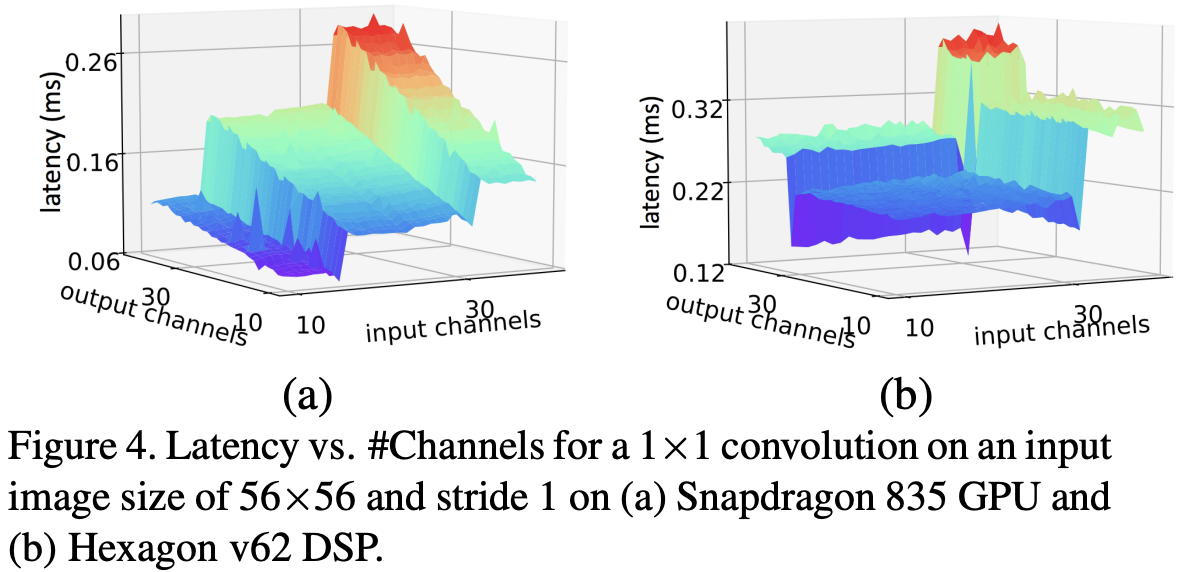• 网络深度的意义CNN 的网络层能够对输入图像数据进行逐层抽象，比如第一层学习到了图像边缘特征，第二层学习到了简单形状特征，第三层学习到了目标形状的特征，网络深度增加也提高了模型的抽象能力。
• 网络宽度的意义：网络的宽度（通道数）代表了滤波器（3 维）的数量，滤波器越多，对目标特征的提取能力越强，即让每一层网络学习到更加丰富的特征，比如不同方向、不同频率的纹理特征等。

## 后续改进-MobileDets

1. FLOPs 低不等于 latency 低，尤其是在有加速功能的硬体 (GPUDSPTPU )上不成立。
2. MobileNet conv block (depthwise separable convolution)在有加速功能的硬件（专用硬件设计-NPU 芯片）上比较没有效率。
3. channel 数的情况下 (如网路的前几层)，在有加速功能的硬件使用普通 convolution 通常会比separable convolution 有效率。
4. 在大多数的硬件上，channel 数为 8 的倍数比较有利计算。
5. DSPTPU 上，一般我们需要运算为 uint8 形式，quantization低精度量化）是常见的技巧。
6. DSPTPU 上，h-Swishsqueeze-and-excitation 效果不明显 (因为硬体设计与 uint8 压缩的关系)。
7. DSPTPU 上，5x5 convolution 比较没效率。

「其他文章」